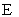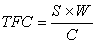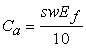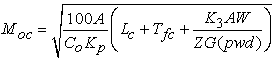﻿ Computer Modeling for Prediction of Implement Field Performance Variables

### Computer Modeling for Prediction of Implement Field Performance Variables

Moayad B. Zaied, Ahmed M. El Naim, Tarig E. MahmoudOPEN ACCESSPEER-REVIEWED

## Computer Modeling for Prediction of Implement Field Performance Variables

Moayad B. Zaied1, Ahmed M. El Naim2,, Tarig E. Mahmoud3

1Department of Agric. Eng., Faculty of Natural Resources and Environmental Studies, University of Kordofan, Elobied, Sudan

2Department of Crop Sciences, Faculty of Natural Resources and Environmental Studies, University of Kordofan, Elobied, Sudan

3Department of Agricultural Economics and Rural Development, Faculty of Natural Resources and Environmental Studies, University of Kordofan, Elobied, Sudan

### Abstract

Size selection of agricultural machinery must necessarily base on anticipated performance and anticipated cost. In field machinery selection, the most pertinent variable is size or capacity of the machine. A computer program was developed in C++ programming language, to predicted implement performance parameters are total field time, theoretical field capacity, effective field capacity and field efficiency for 1.0, 1.5 and 2 m width implement at operation speed of 4, 4.5, 5, 5.5 and 6 km/hr. Program was built, compiled and was then debugged. It was found that as speed and implement width increased, the total field time decreased while theoretical field capacity and effective field capacity increased and field efficiency decreased. The highest field efficiency was 85.5% and it was recorded by implement width of 2 m at 4.5 km/hr speed while the lowest field efficiency was 80.7% and it was recorded by implement width of 1 m 6 km/hr. It was concluded that width of plow found to has higher effect than plow operating speed on increasing the effective field capacity, consequently, the field efficiency.

### At a glance: Figures

1234
Prev Next

• Zaied, Moayad B., Ahmed M. El Naim, and Tarig E. Mahmoud. "Computer Modeling for Prediction of Implement Field Performance Variables." World Journal of Agricultural Research 2.2 (2014): 37-41.
• Zaied, M. B. , Naim, A. M. E. , & Mahmoud, T. E. (2014). Computer Modeling for Prediction of Implement Field Performance Variables. World Journal of Agricultural Research, 2(2), 37-41.
• Zaied, Moayad B., Ahmed M. El Naim, and Tarig E. Mahmoud. "Computer Modeling for Prediction of Implement Field Performance Variables." World Journal of Agricultural Research 2, no. 2 (2014): 37-41.

 Import into BibTeX Import into EndNote Import into RefMan Import into RefWorks

### 1. Introduction

Size selection of machinery must necessarily base on anticipated performance and anticipated cost. In field machinery selection, the most pertinent variable is size or capacity of the machine. Forward speed and power found to affect field capacity and effectiveness of operation .

Witney  indicated that implement might be selected depending on width for getting sufficient capacity so that the needed work to be done within allotted time, and getting the maximum net profit, therefore, selection of width can be estimated as the followsWhere,

EFC = effective field capacity, ha/h.

CF = correction factor.= field efficiency, decimal.= optimum width, m.= forward speed, km/hr.

Measures of agricultural machine performance are the rate and quality, which the operations are accomplished. Rate is an important measure because few industries require such timely operation as agriculture with its sensitivity to quality describing a machine ability to operate without wasted product. Field capacity of an agricultural machine is the rate at which farm operations are accomplished .

The theoretical field capacity of an implement is the rate of field coverage that would be obtained when the machine is performing its function using hundred percent of the time at the rated forward speed and always covering hundred percent of the rated width . Culpin  mentioned equation of theoretical field capacity as followsWhere,

TFC = theoretical field capacity, ha/h.

S = speed, km/hr.

W = implement width, m.

C = constant = 10.

The effective field capacity is the actual rate of performance of land or crop processed in a given time and it can be expressed in area / time or material / time. It was found that the effective field capacity was affected by implement size, Ahmed and Haffar  reported that heavy disc harrow showed higher effective field capacity than light disc harrow. Farmer can increase field capacity by increasing the operating speed or implement width, it would appear that doubling the size or speed would double capacity .

As reported in ASAE EP496  Field efficiency is the ratio between the productivity of a machine under field conditions and the theoretical maximum productivity. Field efficiency accounts for failure to utilize the theoretical operating width of the machine; time lost because of operator capability and habits and operating policy; and field characteristics. Travel to and from a field, major repairs, preventive maintenance, and daily service activities are not included in field time or field efficiency. Field efficiency is not a constant for a particular machine, but varies with the size and shape of the field, pattern of field operation, crop yield, moisture, and crop conditions. The following activities account for the majority of time lost in the field:

i. turning and idle travel;

ii. materials handling;

iii. cleaning clogged equipment;

v. lubrication and refueling (besides daily service);

vi. waiting for other machines.

The effective capacity can be calculated on Area base or material base as followwhere,

Ca = area capacity, ha/h.

S = field speed, km/h.

W = implement working width, m.

Ef = field efficiency, decimal.Where,

Cm = material capacity, t/h,

s = field speed, km/h,

w = implement working width, m,

Ef = field efficiency, decimal,

y = unit yield of the field, t/ha.

The optimum capacity of a machine can be estimated from equation bellowWhere,

Moc = optimum capacity of a machine, ha/h (acre/h);

A = area, ha (acre);

Co = ownership cost percentage, percent;

Kp = unit price function, dollars/ha·h (dollars/acre·h);

Lc = labor cost, dollars/ha (dollars/acre);

Tfc = tractor ownership cost, dollars/ha (dollars/acre);

K3 = timeliness coefficient obtained from ASAE D497, clause 8;

A = area, ha (acre);

Y = yield per area, t/ha (ton/acre);

V = value per yield, dollars/t (dollars/ton);

Z = 4 if the operation can be balanced evenly about the optimum time, and a value of 2 if the operation either commences or terminates at the optimum time; G is expected time available for field work each day, h; pwd is probability of a working day, decimal.

Peterson et al.  found that field efficiency decreased with increasing implement width when field operations were conducted between terraces. Randal et al.  reported that field efficiency decreased with increasing planter width. Field size had little impact on field efficiency. Steichen and Powell  presented a farm ability index for terraced fields and concluded that field efficiency was a function of implement and terrace design.

Field efficiency includes the effect of the time lost in the field and failure to utilize the full width of the machine (Bower, 1985). It is not constant for a particular machine, but varies with the size and shape of the field, pattern of the field operation, crop yield, moisture and crop condition.

Bower  reported that the lost time is the most important factor that affects the field capacity and efficiency of a machine. It may be lost as a result of adjusting or lubricating the machine, break downs, clogging turning at the ends, adding seeds fertilizer or operator personal time . The factors affecting field efficiency were reported by Donnell , as theoretical capacity of the machine, machine maneuverability, field shape, field patterns, field size, yield (if harvesting operation), soil and crop condition and system limitation. Implement type and soil physical condition are important factors affecting the field capacity and efficiency of tillage implement, when soil condition are poor for machine operations forward speed will usually be reduced . Belel and Dahab  found that chisel plow recorded higher values of power requirement, theoretical field capacity and effective field capacity in loose clay soil as compared to disk plow, and moldboard plow.

The objective of the present work is to develop a computer model in C++ programming language to predict theoretical field capacity, effective field capacity and field efficiency of a field operation for implement with different effective widths and different operating speeds.

### 2. Methodology

The variables demonstrated in Table 1 were used as inputs for a program developed in C++ programming language, the program was built, compiled and was then debugged, the flow chart of the program is shown in Figure 1. The predicted implement performance parameters are total field time, theoretical field capacity, effective field capacity, and field efficiency.

### 3. Results and Discussion

Table 2 and Figure 2 showed the performance variables of a plow width 1 m effective, Table 3 and Figure 3 showed the performance variables of a plow width 1.5 m effective width, while Table 3 and Figure 3 showed the performance variables of a plow width 2 m effective width, each operated at speeds of 4 km/hr, 4.5 km/hr, 5 km /hr, 5.5 km/hr and 6 km/hr.

#### Table 3. Plow performance at 1.5 m effective width

From Table 2, Figure 2, Figure 3 and Figure 4 for 1 m implement width, it was demonstrated that at 4 km/hr, the value of total field time was 0.588 hr, theoretical field capacity was 0.400 ha/hr, effective field capacity was 0.340 ha/hr and field efficiency was 85.0%. At 4.5 km/hr, the values of total field time, theoretical field capacity, effective field capacity and field efficiency were 0.530 hr, 0.450 ha/hr, 0.378 ha/hr and 84% respectively. At 5 km/hr the values were 0.483 hr, 0.500 ha/hr, 0.414 ha/hr and 82.8% for total field time, theoretical field capacity, effective field capacity and field efficiency respectively. In case of 5.5 km/hr, the value of total field time was 0.445 hr, theoretical field capacity was 0.550 ha/ha, effective field capacity was 0.449 ha/hr and field efficiency was 81.6%. At 6 km/hr the values of total field time, theoretical field capacity, effective field capacity and field efficiency were 0.413 hr, 0.600 ha/hr, 0.484 ha/hr and 80.7% respectively.

From Table 3, Figure 5, Figure 6 and Figure 7 for 1.5 m implement width, it was demonstrated that at 4 km/hr, the value of total field time was 0.391 hr, theoretical field capacity was 0.600 ha/hr, effective field capacity was 0.511 ha/hr and field efficiency was 85.2%. At 4.5 km/hr, the values of total field time, theoretical field capacity, effective field capacity and field efficiency were 0.352 hr, 0.675 ha/hr, 0.568 ha/hr and 84.1% respectively. At 5 km/hr the values were 0.321 hr, 0.750 ha/hr, 0.623 ha/hr and 83.1% for total field time, theoretical field capacity, effective field capacity and field efficiency respectively. In case of 5.5 km/hr, the value of total field time was 0.296 hr, theoretical field capacity was 0.825 ha/ha, effective field capacity was 0.676 ha/hr and field efficiency was 81.9%. At 6 km/hr the values of total field time, theoretical field capacity, effective field capacity and field efficiency were 0.274 hr, 0.900 ha/hr, 0.729 ha/hr and 81.0% respectively.

In case of Table 4 Figure 8, Figure 9 and Figure 100 for 2 m implement width, it was demonstrated that at 4 km/hr, the value of total field time was 0.293 hr, theoretical field capacity was 0.800 ha/hr, effective field capacity was 0.684 ha/hr and field efficiency was 85.5%. At 4.5 km/hr, the values of total field time, theoretical field capacity, effective field capacity and field efficiency were 0.263 hr, 0.900 ha/hr, 0.759 ha/hr and 84.3% respectively. At 5 km/hr the values were 0.240 hr, 1.00 ha/hr, 0.833 ha/hr and 83.3% for total field time, theoretical field capacity, effective field capacity and field efficiency respectively. In case of 5.5 km/hr, the value of total field time was 0.221 hr, theoretical field capacity was 1.10 ha/ha, effective field capacity was 0.905 ha/hr and field efficiency was 82.3%. At 6 km/hr the values of total field time, theoretical field capacity, effective field capacity and field efficiency were 0.205 hr, 1.20 ha/hr, 0.976 ha/hr and 81.3% respectively.

The results showed that as speed increased the total field time decreased and the theoretical and effective field capacity increased while the field efficiency decreased. It can be concluded that it is not necessary to have a higher field efficiency at higher speed while the width of implement was found to has a noticed effect in improving the field efficiency as shown when the width increased from 1 m to 2 m that the field efficiency increased from 85% to 85.5%.

### 4. Conclusion

A computer model was developed with C++ programming language to predict the field performance parameters of implement with different width operated at different speeds. Width of plow found to has higher effect than plow operating speed on increasing the effective field capacity, consequently, the field efficiency.

According to the results obtained from this research, as speed increased the total field time decreased and the theoretical and effective field capacity increased while the field efficiency decreased. It can be concluded that it is not necessary to have a higher field efficiency at higher speed while the width of implement was found to has a noticed effect in improving the field efficiency.

### References

  Donnell, H. Farm Power and Machinery Management Wiley, Technology & Engineering - 368 pages, 2001.In article  Witney, B. (1988). Choosing and Using Farm Machines. Edinburgh, Land Technology Ltd, pp: 412.In article  Donnell, H. Farm Power and Machinery Management. Wiley, 9 th edition - Technology & Engineering - 363 pages 0813805821, 9780813805825, 1995.In article  Kepner, A. R., Bainer, R. and Barger, L. E. Principles of Farm Machinery, 3 rd Edition,1978, pp: 23-47In article  Cuplin, C. Profitable farm mechanization, 3rd Edition, published by Crosby Lockwood Staples, London, UK, 1976.In article  Ahmed, M. H and Haffar. Comparison of five tillage systems for cotton production in Rahad Scheme. A.M.A,1993, 24 (2): 17-20.In article  ASAE EP 496.2 FEB03 STANDARDS. Agricultural Machinery Management. American Society of Agricultural Engineers, 2003, 367-372.In article  Peterson, C.L., Miller, C.L. Milligan, J.H. and Withers, R.V.. Economic impact of terraces on drylandfarming. TRANSACTIONS of the ASAE, 1981, 24 (4): 951-956.In article CrossRef  Randal, K. Taylor, Mark D. Schrock and Scott A. Staggenborg. Using GPS Technology to Assist Machinery Management Decisions. Paper No. MC01-204. An ASAE Meeting Presentation, 2001.In article  Steichen, J.M. and Powell, G.M.. Measuring farmability of terrace systems.TRANSACTIONS of the ASAE, 1985, 28 (4): 1130-1134.In article CrossRef  Bowers, C.G.J. Southeastern tillage energy data and recommended reporting. T ASAE, 1985, 28 (3), 731-737.In article CrossRef  Belal, M.M and Dahab, M.H. Effect of Soil Condition on a two wheel drive tractor performance, using three types of tillage implements. University of Khartoum, Journal of Agricultural Science, 1997, 5 (2): 1-22.In article